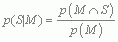# Zach’s facts

Zach’s facts have been extracted from the book to remind you of the key concepts you and Zach have learned in each chapter.

Zach's Facts 7.1 Probability

• The classical probability of an outcome is the theoretical frequency of that outcome divided by the total number of possible outcomes (the sample space).

• The empirical probability of an outcome is the observed frequency of that outcome divided by the total number of observed outcomes (the sample space). It is the same as the relative frequency.

• Probability values vary from 0 (impossible) to 1 (certain), with 0.5 meaning that an event is as likely to happen as it is not to happen. They cannot be negative.

• Probabilities are proportions, and so can be multiplied by 100 and expressed as percentages: 0% = impossible to occur, 50% = as likely to occur as not, 100% = certain to occur.

Zach's Facts 7.2 The standard normal distribution

• The probability density function of a distribution describes the shape of the distribution in terms of the probability of scores occurring.

• If we believe a distribution is shaped like a normal distribution, then we can use the probability density function for the normal distribution to find out the probability of scores for the measure we’ve taken.

• To do this we convert our scores to z-scores so that we can compare them to a standard normal distribution, which is a normal distribution of z-scores (it has a mean of 0 and a standard deviation of 1).

• In doing so, we can calculate the probability that a given score will occur by using tabulated probability values for the standard normal distribution (Section A.1).

Zach's Facts 7.3 Using the standard normal distribution

• The probability density function of the normal distribution can be used to calculate the probability of obtaining scores bigger or smaller than a particular value.

• First convert the score (or scores) to a z-score (see equation 6.1).

• To find the probability of obtaining a score at least as big as a certain value, look up the value of the corresponding z in the table of the standard normal distribution. If the z is positive read the value in the ‘smaller proportion’ column, but if it is negative read the value in the ‘larger proportion’ column.

• To find the probability of obtaining a score as big as a certain value or smaller, look up the value of the corresponding z in the table of the standard normal distribution. If the z is positive read the value in the ‘larger proportion’ column, but if it is negative read the value in the ‘smaller proportion’ column.

• To find the probability of obtaining a score between two values look up the corresponding zs in the table of the standard normal distribution.
• For the smaller z, if it is positive note the value in the ‘larger proportion’ column, but if it is negative note the value in the ‘smaller proportion’ column.

• For the larger z, if it is positive note the value in the ‘smaller proportion’ column, but if it is negative note the value in the ‘larger proportion’ column.

• The probability is 1 minus the values you noted for the two z-scores.

Zach's Facts 7.4 Conditional probability

• Two events are mutually exclusive if it is impossible for them both to happen. For example, you can’t both pass and fail a test, so those outcomes are mutually exclusive.

• Conditional probability is the probability of an outcome, conditional on another outcome happening. For example, you could count how many people survive (S) the probability bridge depending on whether they have Milton helping them (M); the conditional probability p(S|M) is the probability of survival, given Milton’s help. It is calculated as the probability of surviving and having Milton’s help, divided by the probability of having Milton’s help:• Bayes’ theorem expresses conditional probability in terms of the reverse conditional probability and the individual probabilities of the individual events. For example, the probability of surviving, conditional on having Milton’s help, p(S|M), would be expressed as a function of the probability of Milton helping, given that you survived, p(M|S), the probability of Milton helping, p(M), and the probability of survival, p(S):